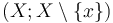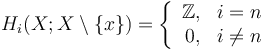# Homology manifold

This article defines a property of topological spaces: a property that can be evaluated to true/false for any topological space|View a complete list of properties of topological spaces

## Definition

Suppose$n$ is a nonnegative integer. A topological space$X$ is said to be a homology manifold of dimension$n$ if it satisfies both the following conditions:

•$X$ is a locally compact space.
• For every point$x \in X$, the homology groups for the pair$(X; X \setminus \{ x \})$ are as follows:$H_i(X; X \setminus \{ x \}) = \left \lbrace \begin{array}{rl} \mathbb{Z}, & i = n \\ 0, & i \ne n\end{array} \right.$

We can similarly define the concept of a homology$G$-manifold of dimension$n$ for any abelian group: instead of looking at the homology groups, we look at the homology groups with coefficients in$G$. The usual definition of homology manifold therefore equals homology$G$-manifold.

## Relation with other properties

### Stronger properties

Property Meaning Proof of implication Proof of strictness (reverse implication failure) Intermediate notions